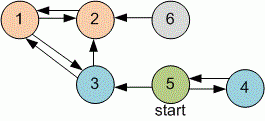Competitions

# The shortest distance

The directed graph is given. Find the shortest distance from the vertex x to other vertices of the graph.

#### Input

First line contains two integers n and x (1n1000, 1xn) - the number of vertices in a graph and the starting vertex. Next n lines contains n numbers - the adjacency matrix of the graph: the i-th line and j-th column contains "1", if the vertices i and j are connected with the edge, and "0", if there is no edge between them. The main diagonal contains zeroes.

#### Output

Print the numbers d1, d2, ..., dn, separated with a space, where di is -1 if there is no path from x to i, or the minimal distance from x to i otherwise.Time limit 2 seconds
Memory limit 128 MiB
Input example #1
6 5
0 1 1 0 0 0
1 0 0 0 0 0
1 1 0 0 0 0
0 0 0 0 1 0
0 0 1 1 0 0
0 1 0 0 0 0

Output example #1
2 2 1 1 0 -1